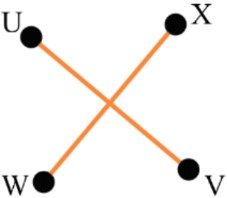# Parallel and perpendicular line segments

### Parallel and perpendicular line segments

In this lesson, we will learn:

• How to measure and draw angles using a protractor
• How to identify whether pairs of lines or line segments are parallel or perpendicular

Notes:

• Protractors are used for measuring and drawing angles: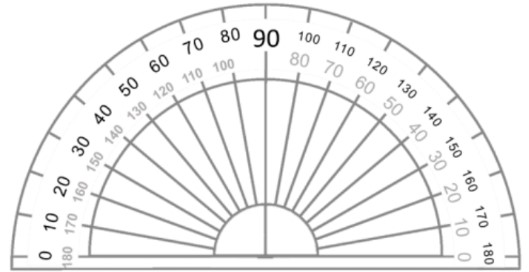• The middle of the flat side of the protractor goes on the vertex of the angle
• There are two scales on the protractor from 0° to 180° (one going the clockwise way, one going the counter-clockwise way)
• Line up one of the zeroes on the protractor with one arm of the angle and start measuring from 0° until you reach the other arm

• Two lines or line segments can either intersect (cross) each other or be parallel. In this section, we will be learning how to identify and draw parallel and perpendicular line segments. Parallel line segments never meet, no matter how far they are extended. Two line segments are perpendicular if they form a 90 degree angle where they cross. Parallel and perpendicular line segments are found everywhere in the world around us. For example, city planners often design roads to be parallel and perpendicular to each other. Along with roads, railroad tracks, lines on notebook paper, and bookcases are parallel.
• When two figures are parallel with each other we can use the symbol: $\parallel$
• When two figures are perpendicular with each other we can use the symbol: $\perp$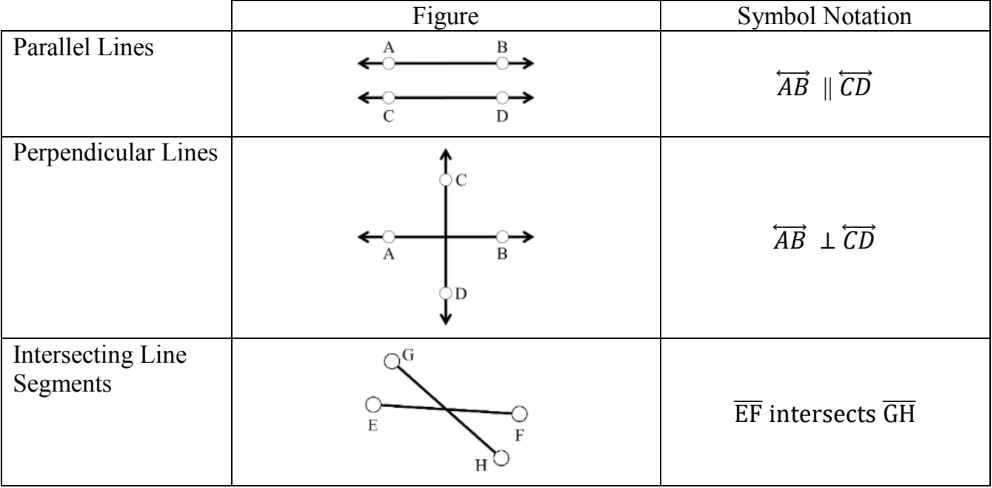#### Lessons

• Introduction
a)
How to use a protractor?

b)
What are parallel lines?

c)
What are perpendicular lines?

• 1.
Are line segments AB and BC parallel or perpendicular?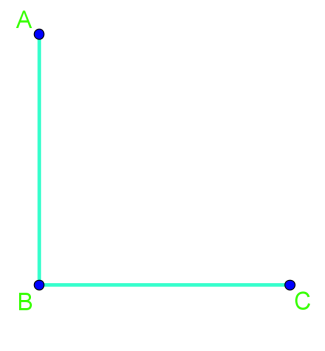• 2.
Below is a line segment, AB.
a)
Draw a line segment that is perpendicular to AB.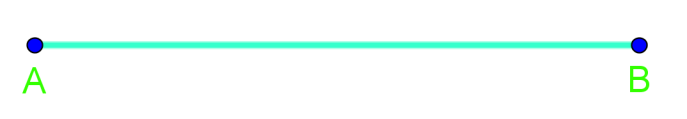b)
Draw a line segment that is parallel to AB.• 3.
Identify the parallel and perpendicular streets in the diagram below.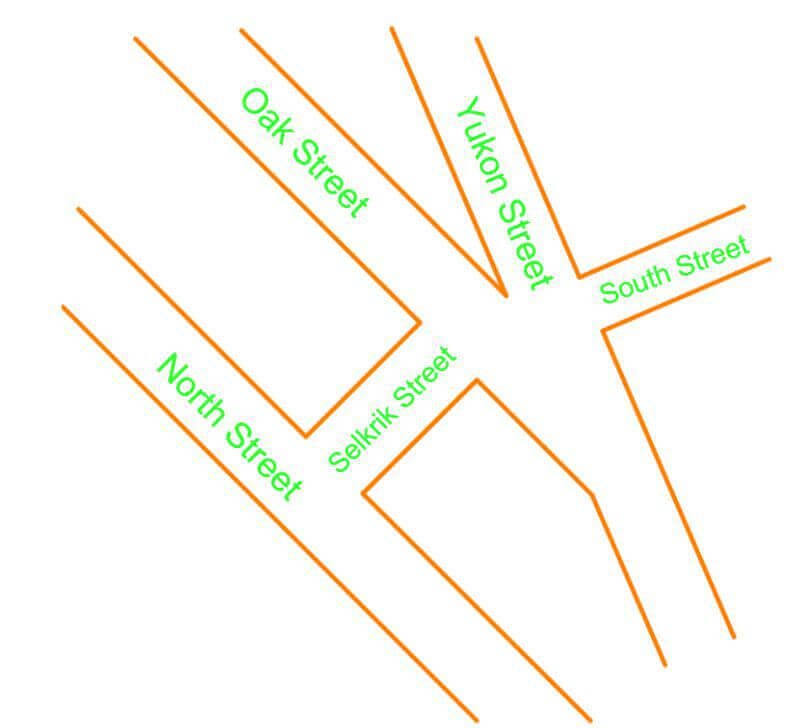• 4.
Parallel or not parallel
Are these line segments parallel? Answer YES or NO.
a)
$\overline{AB} \, \parallel \, \overline{CD}$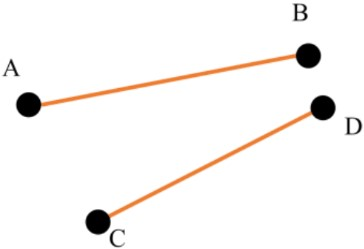b)
$\overline{EF} \, \parallel \, \overline{GH}$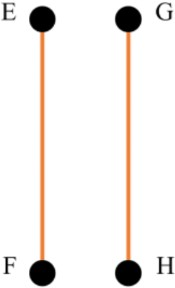c)
$\overline{IJ} \, \parallel \, \overline{KL}$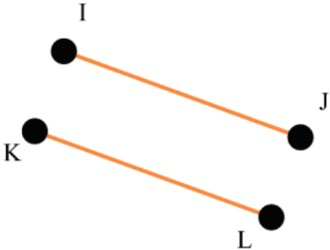• 5.
Perpendicular or not perpendicular
Are these lines perpendicular? Answer YES or NO.
a)
$\overline{MN} \, \perp \, \overline{OP}$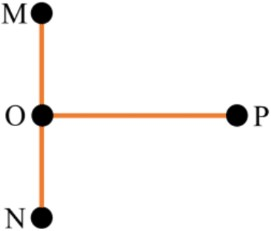b)
$\overline{QR} \, \perp \, \overline{ST}$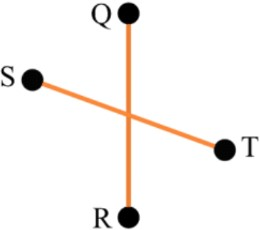c)
$\overline{UV} \, \perp \, \overline{WX}$